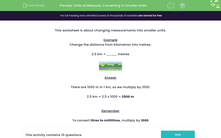# Convert Units of Measurement to Solve Problems

In this worksheet, students will answer a selection of word problems relating to measurements.Key stage:  KS 2

Curriculum topic:   Measurement

Curriculum subtopic:   Convert Units of Measure

Difficulty level:#### Worksheet Overview

For this activity, and indeed for all your maths work way into the future(!), it will be useful to remember the following facts:

Lengths/Distances

100 centimetres (cm) = 1 metre (m)

1,000 metres (m) = 1 kilometre (km)Mass/Weight

1,000 grams (g) = 1 kilogram (kg)Capacity

1,000 millilitres (ml) = 1 litre (l)Looking at those facts above, can you guess what the prefix 'kilo' means?It means 1,000 which makes it easier to remember some of these measurements.

Right then, let's get started and see how we get on!

### What is EdPlace?

We're your National Curriculum aligned online education content provider helping each child succeed in English, maths and science from year 1 to GCSE. With an EdPlace account you’ll be able to track and measure progress, helping each child achieve their best. We build confidence and attainment by personalising each child’s learning at a level that suits them.

Get started